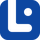# Fractions, Decimals and Percentages

A fraction is a particular form of writing numbers. In this lesson, we will learn how to use them.## What Are Fractions?

Fractions are a way of expressing a part of a whole or a ratio of two numbers. A fraction consists of two numbers separated by a horizontal or diagonal line.

## Why Do We Use Fractions?

Fractions are used to refer to a portion or part of something else but not the whole amount. Fractions are made of an ordinal and a cardinal number.

## Different Parts of a Fraction

Here are the different parts of a fraction:

• numerator: is placed above the dividing line and indicates the parts that are selected. It is always a cardinal number
• dividing line: a horizontal or diagonal bar which separates the parts of a fraction.
• denominator: is placed under the dividing line and represents the total number of equal parts in the whole. It is always an ordinal number.

## Fractions: Types

There are four different types of fractions:

• Unit fractions
• Proper fractions
• Improper fractions
• Mixed fractions

### Unit Fractions

This is when the numerator is '1' and we read the denominator in a singular form.

Fractions Written Form
1/2 One half or a half
1/9 One ninth
1/3 One third
1/4 One forth or a quarter

### Exceptions

1. when the denominator is 2, the fraction is called a half (not a 'second')
2. when the denominator is 4, the fraction is called a quarter (not a 'fourth')

You have half an hour to finish your homework.

I need two halves of watermelon.

'Halves' is the irregular plural form of 'half.'

### Proper Franctions

When the numerator of a fraction is bigger than '1', the denominator is read in plural form.

Fraction Written Form
3/7 Three sevenths
2/3 Two thirds
2/5 Two fifths
6/8 Six eighths

### Improper Fractions

This is when the numerator is bigger than or equal to the denominator.

Fraction Written Form
4/3 Four thirds
5/4 Five fourths
3/4 Three fourths
6/5 Six fifths

### Mixed Fractions

This is when there is a whole number next to a unit or proper fraction. To say the number, we say the whole number first followed by 'and' and finally the fraction number.

6 3/5 Six and three fifths
2 3/4 Two and three fourths
4 1/2 Four and a half
Fraction Written Form
1/2 a half
1/4 a quarter

I need to cut a board that is two and three fourth inches thick.

I walked for four and a half miles this morning.

## Punctuation

When a fraction is used as an adverb or adjective, insert a hyphen between its parts.

a two-thirds decrease (Not 'a two thirds decrease')

We do not use a hyphen when the fraction is used as a noun.

a decrease of two thirds (Not 'a decrease of two-thirds)

### Warning

Do not mix figures and words.

a two-thirds decrease (Not 'a 2 thirds decrease')

## What Are Decimals?

Numbers smaller than one can be shown by decimals. In English, decimal numbers are separated by a decimal point and not a comma.

## How to Read Out Decimals?

the numbers after the decimal point are read out digit by digit, not as a compound number.

Decimal number Written form
0.2 Zero point two
0.25 Zero point two five
1.032 One point zero three two
12.025 Twelve point zero two five
12.006 Twelve point zero zero six

### Tip!

Remember that in English, we use a decimal point for decimal numbers and a comma to separate thousands.

12.025

twelve point zero two five

12,025

twelve thousand and twenty five

### Warning

We never use hundred and thousand after the decimal point.

## What are Percentages

Percentages are easy and commonly used in our everyday conversations. A percentage is a number or ratio that expresses a fraction of 100 with the symbol '%'. To read them, we say the number and then the word percent. Check out some examples:

Percentages How to Say
2% two percent
35% thirty-five percent
50% fifty percent
70% seventy percent
90.5% ninety point five percent
100% a/one hundred percent

We can also turn percentages into decimal numbers:

Percentage In decimal number
50% 0.5
24% 0.24
2.4% 0.024

### You might also like

#### Ordinal Numbers

Imagine that you are on a list and you want to know where you are standing. To refer to that number, we use 'ordinal numbers.'

#### Roman Numerals

The ancient numbers that are still being used, we all have definitely seen them, let's learn them properly now.

#### When to Spell Out Numbers

There are many types of ways to say a number but do you know when and where to use each of them? In this lesson, we will discover more about numbers.

#### Measurements

The act of telling the size, length, weight, etc, of something, is called measurement which is all about numbers and fractions.

#### Expressing Dates

Telling the date is one of the most common subjects in our daily life. In this lesson, we will learn how to express the date of something.

#### Expressing Time

Expressing time is not just about time and numbers. In this lesson, we will learn how to tell time and learn more about it.